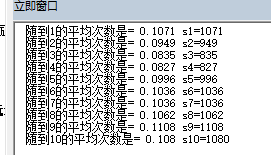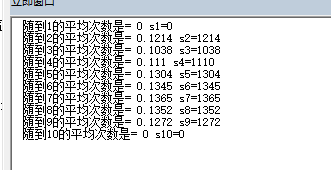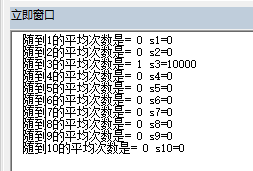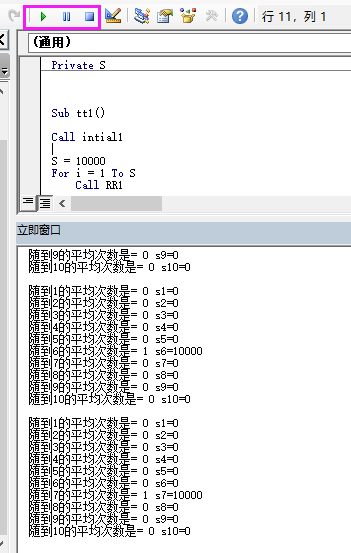• 'Dim min As Integer '定义随机数的最小值 'Dim max As Integer '定义随机数的最大值 '限制只产生500个，VBA的速度问题 Public Function CreateRND(ByVal min As Integer, _
<span style="font-family: Arial, Helvetica, sans-serif;"></span><pre name="code" class="vb">'Dim min As Integer                             '定义随机数的最小值
'Dim max As Integer                             '定义随机数的最大值
'限制只产生500个，VBA的速度问题
Public Function CreateRND(ByVal min As Integer, _
ByVal max As Integer) As Integer()

Dim a_size As Integer

a_size = max - min

If a_size > 500 Or max - min + 1 < a_size Then: Exit Function             '限制只产生500个，VBA的速度问题
'如果最大值和最小值的差小于数组大小数值就什么也不做

Dim arr() As Integer                            '定义数组
ReDim arr(a_size)                               '更改数组大小
Dim flag As Boolean                             '定义标志变量，用来判断是否有重复值

Randomize (Now())                               '用当前时间生成随机数种子

For i = 0 To a_size                             '循环生成随机数

Do
arr(i) = Rnd() * (max - min) + min      '生成随机数

flag = False

For j = 0 To (i - 1)                    '循环判断当前的随机数是否和前面生成的随机数相同，如果相同就重新生成

If (arr(i) = arr(j)) Then
flag = True
End If

Next

Loop While flag

'循环中不断生成随机数，直到不重复为止

Next

CreateRND = arr

End Function


调用方法：

    Dim arr() As Integer
arr = CreateRND(10034, 0, 10034)



展开全文• <p>VBA自定义一个函数Unirands（a，b，n，m）随机产生【a，b】内的随机数填写到n✖️m的矩阵中 比如说unirands（...产生500-510的随机数 填写到3✖️3的矩阵中</p>windows
• 用EXCEL生成随机数 用VNA生成随机数 生成1-10的随机数 p1 = Int(1 + 10* Rnd()) ---这里正确，刚好10=10-1+1 p1 = Int(1 + (10-1+1) * Rnd()) --总是正确 如果生成 2-9之间的随机数 p2 = Int( 2 + 9*...

1用EXCEL生成随机数
rand()  也是 [0,1）randbetween(a,b)   是  [a,b]

随机，randbetween() 只能随机整数，如果是要小数，那就先乘100，随机完之后再除100这样处理
randbetween(a,b)   是  [a,b]
RANDBETWEEN(1000*G49,1000*H49)/1000

2用VBA生成随机数
官方解释

为了生成某个范围内的随机整数，可使用以下公式： Int((upperbound - lowerbound + 1) * Rnd + lowerbound) 这里，upperbound 是随机数范围的上限，而 lowerbound 则是随机数范围的下限。 注意 若想得到重复的随机数序列，在使用具有数值参数的 Randomize 之前直接调用具有负参数值的 Rnd。使用具有同样 number 值的 Randomize 是不会得到重复的随机数序列的。

rnd 是左闭右开区间   [0,1)如果要生成 a~b之间的随机数，一般是int(a+ (b-a+1)*rnd)因为一般都是要在整数之间随机 要用 int()

2.1 例子
生成1-10的随机数
p1 = Int(1 + 10* Rnd())              ---这里正确，刚好10=10-1+1p1 = Int(1 + (10-1+1) * Rnd())     --总是正确如果生成 2-9之间的随机数
p2 = Int( 2 + 9* Rnd() )              --错误的p2 = Int(2 + (9-2+1) * Rnd())     ----正确2.2 还玩了下其他的

rnd(-1)
用永远生成相同的随机数相当于固定随机数种子了？这样的应用情景是？rnd(0)
虽然随机序列都一样，但和rnd(-1) 不同每次连续随机，也是一样的随机数种子，总是用上次的但是如果先停止，再重新开始，就是用下一个随机种子，会和以前的不同3 下面是测试随机数的代码
Private p1
Private s1, s2, s3, s4, s5, s6, s7, s8, s9, s10
Private S

Sub tt1()

Call intial1

S = 10000
For i = 1 To S
Call RR1
Call SS1
'    Debug.Print p1
Next

Call SS2
End Sub

Function intial1()

s1 = 0
s2 = 0
s3 = 0
s4 = 0
s5 = 0
s6 = 0
s7 = 0
s8 = 0
s9 = 0
s10 = 0

End Function

Function SS2()
Debug.Print "随到1的平均次数是= " & s1 / S & " s1=" & s1
Debug.Print "随到2的平均次数是= " & s2 / S & " s2=" & s2
Debug.Print "随到3的平均次数是= " & s3 / S & " s3=" & s3
Debug.Print "随到4的平均次数是= " & s4 / S & " s4=" & s4
Debug.Print "随到5的平均次数是= " & s5 / S & " s5=" & s5
Debug.Print "随到6的平均次数是= " & s6 / S & " s6=" & s6
Debug.Print "随到7的平均次数是= " & s7 / S & " s7=" & s7
Debug.Print "随到8的平均次数是= " & s8 / S & " s8=" & s8
Debug.Print "随到9的平均次数是= " & s9 / S & " s9=" & s9
Debug.Print "随到10的平均次数是= " & s10 / S & " s10=" & s10

End Function

Function RR1()

Randomize
p1 = Int(1 + (10) * Rnd())
'Debug.Print p1
End Function

Function SS1()

Select Case p1
Case Is = 1
s1 = s1 + 1
Case Is = 2
s2 = s2 + 1
Case Is = 3
s3 = s3 + 1
Case Is = 4
s4 = s4 + 1
Case Is = 5
s5 = s5 + 1
Case Is = 6
s6 = s6 + 1
Case Is = 7
s7 = s7 + 1
Case Is = 8
s8 = s8 + 1
Case Is = 9
s9 = s9 + 1
Case Is = 10
s10 = s10 + 1
End Select

End Function



展开全文• VBA编程实现不重复随机数输出。VBA里的随机函数是RND，在工作表中随机函数是RAND，一字之差，可要记好了。RND取值范围是[0,1)，意思是0和1之间的一个随机数，包含0，但不包含1。 1、用法 语法：Rnd[(number)] 　如果...
作者：iamlasong
VBA编程实现不重复随机数输出。VBA里的随机函数是RND，在工作表中随机函数是RAND，一字之差，可要记好了。RND取值范围是[0,1)，意思是0和1之间的一个随机数，包含0，但不包含1。
1、用法
语法：Rnd[(number)] 　　如果 number 的值是 Randomize 生成 　　小于 0 ，每次都使用 number 作为随机数种子得到的相同结果。 　　大于 0 ，以上一个随机数为种子产生下一个随机数。 　　等于 0 ，产生与最近生成的随机数相同的随机数。 　　省略， 以上一个随机数为种子产生下一个随机数（同大于0）。 说明： 　　Rnd 函数返回小于 1 但大于或等于 0 的值。 　　number 的值决定了 Rnd 生成随机数的方式。 　　对最初给定的种子都会生成相同的数列，因为每一次调用 Rnd 函数都用数列中的前一个数作为下一个数的种子。 　　在调用 Rnd 之前，先使用无参数的 Randomize 语句初始化随机数生成器（若带参数，则产生由参数对应的一个特定序列的随机数），该生成器具有根据系统计时器得到的种子。如果不使用Randomize 语句，那么每次执行程序时产生的随机数序列是相同的。
Rnd 后面跟一个负数时，同样的参数可以得到完全相同的两个序列，例如，rnd -1执行后用rnd取1000个随机数，然后再执行rnd -1，然后再用rnd取1000个随机数，这1000个随机数和前面1000个完全相同。为了得到不同的序列，可以用不同的负数，也可以在rnd -1后面执行Randomize number。注意，要得到相同的序列，两次Randomize后面的number必须相同。这种方法用途之一就是用于加密和解密。　　关于产生相同的随机序列总结一下：1、Rnd(-1)后取随机数与再次执行Rnd(-1)取的随机数相同；2、Rnd(-1)后取随机数与再次执行Rnd(-2)取的随机数不同；3、Rnd(-1)和Randomize 1后取随机数与再次执行Rnd(-1)和Randomize 1取的随机数相同；4、Rnd(-1)和Randomize 1后取随机数与再次执行Rnd(-1)和Randomize 2取的随机数不同。　　为了生成某个范围内的随机整数，可使用以下公式： 　　Int((upperbound - lowerbound + 1) * Rnd + lowerbound) 　　这里，upperbound 是随机数范围的上限，而 lowerbound 则是随机数范围的下限。 　　注意：若想得到重复的随机数序列，在使用具有数值参数的 Randomize 之前直接调用具有负参数值的 Rnd。使用具有同样 number 值的 Randomize 是不会得到重复的随机数序列的。
2、无重复随机数算法一
这是最简单的算法，每产生一个随机数，就和已有的比较，如果已经存在，则重新产生。比较适合从一个大范围里面抽出一小部分数据，比如，从题库中抽取试题。

' 产生20个1-100之间的不重复随机数
Public Sub RndNumberNoRepeat1()

Dim RndNumber, temp(20), i, k, Maxrec As Integer

Randomize (Timer)           '初始化随机数生成器
Maxrec = 100

' 从A21开始输出随机数
k = 0
Do While k < 20
RndNumber = Int(Maxrec * Rnd) + 1
temp(k) = RndNumber
Cells(k + 21, 1) = RndNumber
For i = 0 To k - 1
If temp(i) = RndNumber Then Exit For
Next i
If i = k Then k = i + 1
'MsgBox "随机数：" & RndNumber
Loop

End Sub


3、无重复随机数算法二
这个算法比较巧妙，需要细细体会，才能知道真谛。这个算法不会重复产生随机数，但需要一个占位数组。比较适合输出范围之内所有数值的场合，比如，随机发牌。
' 产生20个1-100之间的不重复随机数
Sub RndNumberNoRepeat2()
Dim RndNumber, TempArray(99), i As Integer
Randomize (Timer)           '初始化随机数生成器
For i = 0 To 99            '产生包含1－100的不重复的随机数列
TempArray(i) = i
Next i
For i = 99 To 80 Step -1
RndNumber = Int(i * Rnd)
'从A21开始输出这些数字
Cells(120 - i, 1) = TempArray(RndNumber) + 1
TempArray(RndNumber) = TempArray(i)
Next i
End Sub

4、无重复随机数算法三
这个算法使用字典对象完成去重复，和第一个算法差不多，但程序看上去简洁一点，嗯，起码新颖一点。

' 产生20个1-100之间的不重复随机数
Sub RndNumberNoRepeat3()
Dim d As Object   'New Dictionary
Dim s As Integer

Randomize (Timer)           '初始化随机数生成器
Set d = CreateObject("Scripting.Dictionary")

Do Until d.Count = 20
s = Int(Rnd * 100 + 1)
d(s) = ""
Loop
[a21].Resize(d.Count, 1) = Application.Transpose(d.Keys)
End Sub

附：VBA中Dictionary对象使用小结 Dim dict ' 创建Dictionary Set dict = CreateObject("Scripting.Dictionary") ' 增加项目 dict.Add "A", 300 dict.Add "B", 400 dict.Add "C", 500 ' 统计项目数 n = dict.Count ' 删除项目 dict.Remove ("A") ' 判断字典中是否包含关键字 dict.exists ("B") ' 取关键字对应的值，注意在使用前需要判断是否存在key，否则dict中会多出一条记录 Value = dict.Item("B") ' 修改关键字对应的值,如不存在则创建新的项目 dict.Item("B") = 1000 dict.Item("D") = 800 ' 对字典进行循环 k = dict.keys v = dict.Items For i = 0 To dict.Count - 1 key = k(i) Value = v(i) MsgBox key & Value Next ' 删除所有项目 dict.Removeall

实例：

Sub 宏1() Set dic = CreateObject("Scripting.Dictionary") '字典 For i = 1 To 10000 If Not i Like "*4*" Then dic.Add i, "" '如果不包含“1” End If Next Range("a2").Resize(dic.Count, 1) = Application.WorksheetFunction.Transpose(dic.keys) '从A2单元开始向下放置 End Sub ========================================================================= 又 Tranpose工作表函数的用法实例 '把一行多列的二维数组转换成一维数组 Sub test() Dim arr, arrt arr = Range("a1:j1") arrt = WorksheetFunction.Transpose(WorksheetFunction.Transpose(arr)) Stop End Sub 首先看看TRANSPOSE函数的基础用法。官方帮助说明，TRANSPOSE函数可返回转置单元格区域，即将行单元格区域转置成列单元格区域，反之亦然。 　　TRANSPOSE函数语法是：TRANSPOSE(array) 　　Array参数是需要进行转置的数组或工作表上的单元格区域。所谓数组的转置就是，将数组的第一行作为新数组的第一列，数组的第二行作为新数组的第二列，以此类推。


展开全文算法
• VBA生成和为定值的随机数 最近完成了一个VBA小程序，生成和为定值的一系列随机数 在A1:D4区域生成随机数，每行的和等于E列的值 首先我们需要引入第一个函数，计算A1:D4的和 Application.Sum(Range(“A1:D1”)) ...
VBA生成和为定值的随机数
最近完成了一个VBA小程序，生成和为定值的一系列随机数在A1:D4区域生成随机数，每行的和等于E列的值 首先我们需要引入第一个函数，计算A1:D4的和 Application.Sum(Range(“A1:D1”)) Range(“A1:D1”)是对单元格A1:D4的引用（也可以用[A1:D4]代替），可以表示值range().value，也可以表示单元格的宽和高等属性 我们加入循环语句，条件为A1:D4的和是否等于E1 Do Until Application.Sum(Range(“A1:D1”)) = Cells(1, 5) 我们再加入一个随机数函数Randomize，Rnd()，用For循环遍历A1:D4中每个元素 Randomize For Each r In Range(“A1:D1”) r.Value = Int(Rnd() * 10) + 1 Next 这样第一行的条件随机数就完成了，想看生成三行随机数代码的同学请点下面链接 VBA源文件
展开全文• 背景：用PPT做一个选号的界面，按开始便不断地产生随机数（1-24），按停止则显示一个随机数。条件：1、24个数字分为6组第一组：1，2，3，4第二组：5，6，7，8第三组：9，10，11，12第四组：13，14，15，16第五组：17...
• Rnd函数在产生随机数的时候，并不bai是真正的随机，而是通过一定的公式计算产生的随机，现在我们把那个计算产生随机数的公式叫做f(x)，第一个x取值为1 那么便会产生第一个随机数f(1)，第2个随机数是在第一个随机数...
• 产生两个及更多范围的随机数。可以用于制作一份随机数据或随机表单。一共讲解了RANDBETWEEN()、RAND()、CHOOSE()、CHAR()、Round()、Trim()、Chr()、Str()、DataSerial()、TimeSerial()、Foramt()这11个Excel函数的...excel
• Sub 随机挑选演示程序1() Dim arr Dim x As Integer, num As Integer, k As Integer Range("c1:c10") = "" Range("a1:a10") = Application.Transpose(Array("A", "B", "C", "D", "E", "F", "G", "H", "I", "J"))...
• Randomize (Timer) '初始化随机数生成器 Maxrec = 100 ’ 从A21开始输出随机数 k = 0 Do While k RndNumber = Int(Maxrec * Rnd) + 1 temp(k) = RndNumber Cells(k + 21, 1) = RndNumber For i = 0 To k -...
• 　在调用 Rnd 之前，先使用无参数的 Randomize 语句初始化随机数生成器（若带参数，则产生由参数对应的一个特定序列的随机数），该生成器具有根据系统计时器得到的种子。如果不使用Randomize 语句，那么每次执行程序...
• 实际上，是很多使用Excel 的朋友有这样的需求，希望能产生一些随机数，大家知道可以用Rand函数来产生它，但是这个函数是易失的，就是说会不断地计算。朋友们可能希望第一次让它随机产生，而后就固定了。所以，这就...
• vba点击开始按钮 在控件中变化显示数字 点击停止不再变化 ，并显示获得的数字
• j = rest - (rest \ 62) * 62 + 1 'vba 角标是从1开始，不是从0开始 retStr = retStr + arr(j) rest = rest \ 62 Wend Convert10To62 = StrReverse(retStr) End Function   转载于:...
• 电子表格中RAND()函数的取值范围是0到1，公式如下： =RAND()   如果取值范围是1到2，公式如下： =RAND()*(2-1)+1 ...生成一随机数，并且使之不随单元格计算而改变，可以在编辑栏中输入“=RAND
• 我们可以计算出累积分布函数的逆函数： 如果要生成符合上述概率密度函数的三角分布，则需要： 举例说明如下： 有一个三角分布，a=1, b=25, c=5,我们希望生成1000个随机数，服从三角分布，则在Excel中可以这样定义...三角分布
• 循环控制产生随机数和累加的次数，跳出循环后输出结果。 程序流程图 源程序代码 import java.util.Random;   import javax.swing.*;  public class Sjxj {   public static void main...
• VBA里 变量 a 字符串 “a” 函数 func() 数组 array() 数组和函数形式很像，所以需要实现定义清楚，否则不好区分 但是VB里，index用 () 而不是一般语言的 []   Sub t3() Dim arr3(3)...
•C++
• 内容提要：文章提供三个vba代码生成不重复随机数的案例供学习。对Excel感兴趣的朋友可加Excel学习交流群：284029260（www.itblw.com） vba不重复随机数案例一： 下面的代码，将在A1：A5000单元格产生1-5000的不...Excel公式 Excel函数 Excel教程 Excel表格
• '生成m到n之间的随机整数 Function RandBetween_VBA(ByVal m As Long, ByVal n As Long) Randomize RandBetween_VBA = Int(Rnd * (n - m + 1) + m) End Functionrandom
•  使用过随机数的程序员都知道在程序中并不能够实现的真正的完全的随机数函数。随机数函数产生的是通过公式计算出来的...基于产生随机数的原理，两次调用随机数后产生的随机数序列将是一样的，显然，这不是我们的期望
• 在多个指定区间内生成随机数，且随机数总和固定算法 一、介绍 最近项目上遇到了一个需求就是在多个指定区间内生成总和恒定的随机数。 示例：在[1-3]、[4-20]、[24-100]区间上分别生成一个随机数且要求随机数总和为40...java 算法
• 这是应用“序列数交换法”产生随机数的演示程序。通过点击几个键你就可以得到唯一的真随机数组。 详见：http://blog.csdn.net/sjd163/archive/2007/01/18/1486430.aspx
• 我们通过随机数函数Rnd结合for循环嵌套来实现生成十个不重复的随机数，代码如下：   Option Explicit Dim a(9) As Integer, i As Integer, j As Integer ‘定义数组及循环变量 Dim Temp As Integer ’定义一...VB编程 编程 高级编程语言
• 代码： package 第8章_集合框架; import java.util.ArrayList; public class ShuZu { public static void main(String[] args) { ... //随机生成一个1-100的随机数，循环10次，总共生成10个。 /java
• 利用json中键唯一的特性var arr = [];var json = {};for(var i = 0;i<50;i++){ var rand = Math.round(Math.random()*100); if(!json[rand]){ arr.push(rand); json[rand] = 1; }else{ i--;...
• ## VBA教学视频

千次阅读 2018-11-26 14:53:00
全民一起VBA视频笔记 基础篇： 视频地址 https://study.163.com/course/courseLearn.htm?courseId=1003088001#/learn/video?lessonId=1003503026&amp;amp;amp;amp;amp;amp;courseId=1003088001 子过程 无......